# Multivariable calculus

## Introduction

So far in A-level maths you've dealt mainly with functions like $$y=f(x)$$

where the variable $y$ is equal to some function of one variable $x$. Such expressions can be differentiated with respect to this one independent variable $$\frac{dy}{dx} = f'(x)$$

In FP3 we deal with functions of multiple variables, sketch what they look like in three dimensions, and analyse their gradients and behaviours.

## Three-dimensional surfaces

The function $y=f(x)$ represents a curve in two dimensions.

Geometrically a function expressed by $z=f(x,y)$ represents a surface in three dimensions, where $z$ is the third dimension in Cartesian three-dimensional space.

This is the surface $z = 2x + 3y$, a flat plane.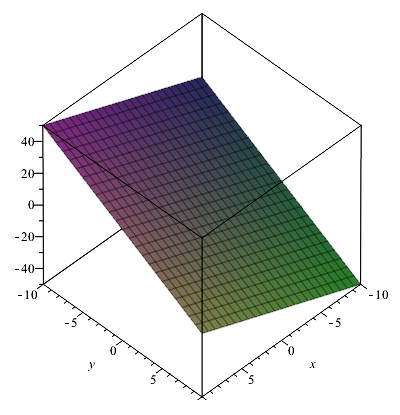You can take sections and contours of this surface to see how 2D and 3D space relate to each other. For example if you set $z=2$, then the surface becomes $$2 = 2x + 3y \Rightarrow y = \frac{2}{3}\left(1-x\right)$$

which looks like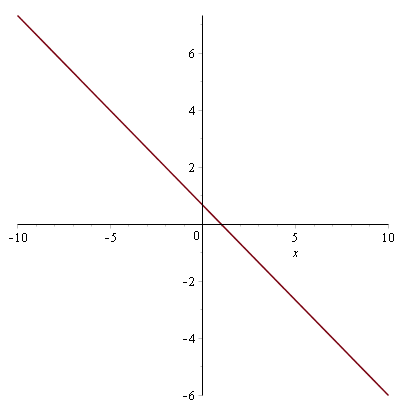Indeed if you compare this to the 3D graph it has similar decreasing behaviour as $x$ increases while $z$ is held constant.

This is a contour plot of the same surface. Since the $x$ and $y$ in $f(x,y)$ are linear the contours aren't particularly interesting. They're just straight lines.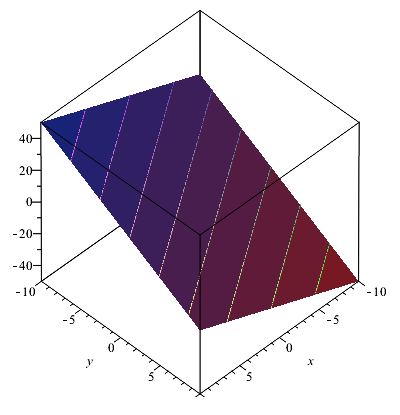In two dimensions the contour plot gives another perspective.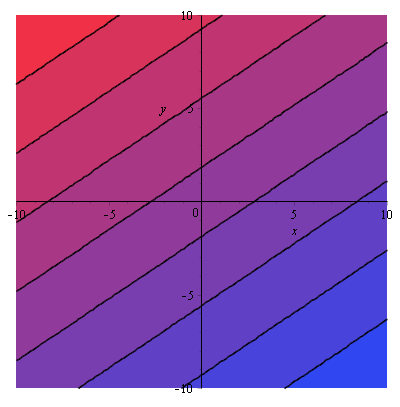A much more interesting surface is $z=x^2-y^3$. It is non-linear and therefore has a much 'curvier' shape.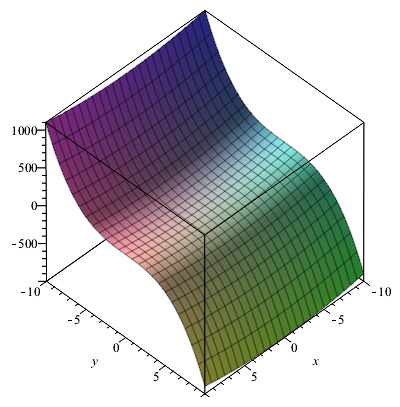Its contour plot shows the changes in gradient across the surface.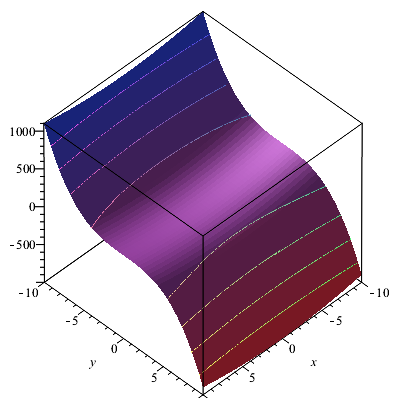In two dimensions the contour plot looks like this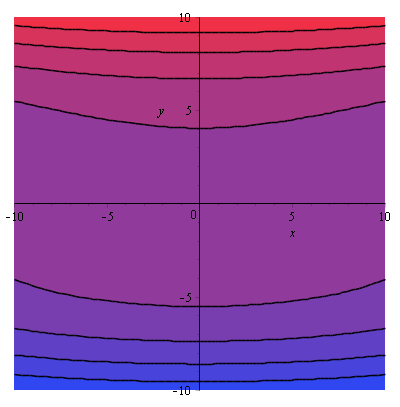## Partial derivatives

A partial derivative of a multivariable function is a derivative with respect to one of its variables, holding the others constant.

For a function $z=f(x,y)$ the partial derivative of $z$ with respect to $x$ is denoted $$\frac{\partial z}{\partial x}$$

A partial derivative of $f(x,y)$ is often denoted in several other ways too $$\frac{\partial f}{\partial x} = f_x = \partial_x f = \frac{\partial}{\partial x}f$$

Take the function $z=xy-\frac{2}{x+y}$. This function is too complicated to differentiate using the conventional methods you learned so far in A-level maths, but you can analyse its behaviour as well as the 3D surface it represents using partial derivatives.

Every point on the surface has infinitely many tangent lines. When you take a partial derivative, you treat all the other variables as constant. That means you treat then just as if they were numbers. So let's the find the partial derivatives

$$\frac{\partial z}{\partial x} = y+\frac{2}{\left(x+y\right)^2}$$

and similarly

$$\frac{\partial z}{\partial y} = x+\frac{2}{\left(x+y\right)^2}$$

### Example

Q) Find the partial derivatives of $z=\sin(x^2 +y)$ with respect to $x$ and $y$.

A) By the chain rule $$\frac{\partial z}{\partial x} = 2x\cos(x^2 +y)$$ and $$\frac{\partial z}{\partial y} = \cos(x^2 +y)$$

### Example

Q) Find the partial derivatives of $z=x\left(x-y\right)^3+3x$ with respect to $x$ and $y$.

A) Using the product rule and the chain rule \begin{align} \frac{\partial z}{\partial x} &= 3x\left(x-y\right)^2+\left(x-y\right)^3+3 \\ &= \left(x-y\right)^2\left(3x+x-y\right)+3 \\ &= \left(x-y\right)^2\left(4x-y\right)+3 \end{align}

and using the chain rule $$\frac{\partial z}{\partial y} = -3xy\left(x-y\right)^2$$

## Stationary points in three dimensions

In C1 and C2 you learned that to find the stationary points of a function $y=f(x)$ you needed to set $$\frac{dy}{dx} = 0$$

and solve for $x$. This told you the points where the gradient was zero. We can do the same thing for surfaces in three dimensions. However instead of the above condition, we need to find points $(x,y,z)$ such that $$\frac{\partial z}{\partial x} = \frac{\partial z}{\partial y} = 0$$

which makes sense since we're looking for points that are 'flat' in three dimensions. Remember that each stationary point must satisfy both $\frac{\partial z}{\partial x}=0$ and $\frac{\partial z}{\partial y}=0$ at the same time.

### Example

Q) Find the stationary points of the surface $z=\sin(x^2 +y)$.

A) We found that the partial derivatives were \begin{align} \frac{\partial z}{\partial x} &= 2x\cos(x^2 +y) \\[0.7em] \frac{\partial z}{\partial y} &= \cos(x^2 +y) \end{align}

Equating both of them to zero and subtracting one from the other $$\frac{\partial z}{\partial x} - \frac{\partial z}{\partial y} = \left(2x-1\right)\cos(x^2 +y) = 0$$

Therefore either $x=\frac{1}{2}$ or $\cos(x^2 +y) = 0$. This means that stationary points occur along the line $x=\frac{1}{2}$ and also on the lines $x^2+y=n\frac{\pi}{2}$ for $n\in\mathbb{Z}$.

### Example

Q) Find the stationary points of the surface $z=x^2+3y$.

A) The partial derivatives are $$\frac{\partial z}{\partial x} = 2x ~\textrm{ and }~ \frac{\partial z}{\partial y} = 3$$

Equating both of them to zero and subtracting one from the other $$\frac{\partial z}{\partial x} - \frac{\partial z}{\partial y} = 2x-3 = 0$$

Therefore stationary points occur along the line $x=\frac{3}{2}$.

Surfaces in three dimensions can also be expressed in the form $$f(x,y,z)=c$$ where $c$ is a constant. Here's an example, $-4x-y^2+z^2=3$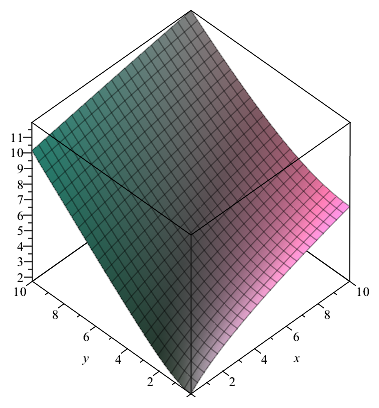Then $\textbf{grad}f$ or $\nabla f$ is a vector function that always points in the direction of greatest increase of $f$ at each particular point. In the context of surfaces it is also called the gradient. The magnitude of $\nabla f$ is the value of the steepest slope. It is calculated as $$\nabla f = \left(\frac{\partial f}{\partial x},\frac{\partial f}{\partial y},\frac{\partial f}{\partial z}\right)$$

In the special case where $f(x,y,z)=c$ can be expressed in the form $z=g(x,y)$ then $$\nabla f = \left(\frac{\partial g}{\partial x},\frac{\partial g}{\partial y},-1\right)$$

For the above example $$\nabla f = \left(-4,-2y,2z\right)$$

and the value of the steepest slope at each point is the norm of the gradient, since it's a vector $$\left\Vert\nabla f\right\Vert = \sqrt{\left(-4\right)^2+\left(-2y\right)^2+\left(2z\right)^2} = 2\sqrt{4+y^2+z^2}$$

### Tangent planes and normal lines

One application of partial derivatives and the surface gradient is finding tangent planes and normal lines to points on surfaces. Recall from AS-level maths that to find a tangent line to a point $(x_0,y_0)$ on a function in two dimensions, where the gradient at that point is $m$, sub the values into $$y - y_0 = m\left(x-x_0\right)$$

In three dimensions, the equation for a plane tangent to a point $(x_0,y_0,z_0)$ on a surface $f(x,y,z)=c$ is made up of the values of the partial derivatives at the point $$f_x(x_0,y_0,z_0)\left(x-x_0\right)+f_y(x_0,y_0,z_0)\left(y-y_0\right)+f_z(x_0,y_0,z_0)\left(z-z_0\right) = 0$$

### Example

Q) Find the tangent plane to the point $(1,3,1)$ on the surface $(x+z)^2+y+\sqrt{z}=2$.

A) Let $f(x,y,z)=(x+z)^2+y+\sqrt{z}$. The partial derivatives are

\begin{align} f_x &= 2x+2z \Rightarrow f_x(1,3,1) = 4 \\ f_y &= 1 \\ f_z &= 2x+2z+\frac{1}{2\sqrt{z}} \Rightarrow f_z(1,3,1) = \frac{9}{2} \\ \end{align}

Therefore the equation of the tangent plane is $$4\left(x-1\right)+\left(y-3\right)+\frac{9}{2}\left(z-1\right) = 0$$ $$\Rightarrow 4x+y+\frac{9}{2}z=\frac{23}{2}$$

The normal line to a point $(x_0,y_0,z_0)$ on a surface $f(x,y,z)=c$ is a straight line starting from the point and going in the same direction as $\nabla f$ at that point. The line is expressed as $$\mathbf{r}(\lambda) = (x_0,y_0,z_0)+ \lambda\nabla f(x_0,y_0,z_0)$$

### Example

Q) Find the equation of the normal line to the point $(2,0,-1)$ on the surface $\left(\frac{x+y}{z}\right)^2 = 0$.

A) Let $f(x,y,z)=\left(\frac{x+y}{z}\right)^2$. The partial derivatives are

\begin{align} f_x &= \frac{2x\left(x+y\right)}{z^2} \Rightarrow f_x(2,0,-1) = 8 \\ f_y &= \frac{2y\left(x+y\right)}{z^2} \Rightarrow f_x(2,0,-1) = 0 \\ f_z &= -\frac{2\left(x+y\right)}{z^3} \Rightarrow f_z(2,0,-1) = 4 \\ \end{align}

Therefore the equation of the normal line is $$\mathbf{r}(\lambda) = (2,0,-1) + (8,0,4)\lambda = (2+8\lambda,0,4\lambda-1)$$

### Example

Q) Find the equations of the tangent plane and the normal line to the point $(-1,-1,\frac{\pi}{3})$ on the surface $\sin(xyz)=1$.

A) Let $f(x,y,z)=\sin(xyz)$. The partial derivatives are

\begin{align} f_x &= yz\cos(xyz) \Rightarrow f_x(-1,-1,\frac{\pi}{3}) = -\frac{\pi}{6} \\ f_y &= xz\cos(xyz) \Rightarrow f_x(-1,-1,\frac{\pi}{3}) = -\frac{\pi}{6} \\ f_z &= xy\cos(xyz) \Rightarrow f_z(-1,-1,\frac{\pi}{3}) = \frac{1}{2} \\ \end{align}

Therefore the equation of the tangent plane is $$-\frac{\pi}{6}\left(x+1\right)-\frac{\pi}{6}\left(y+1\right)+\frac{1}{2}\left(z-\frac{\pi}{3}\right) = 0$$ $$\Rightarrow -\frac{\pi}{6}x-\frac{\pi}{6}y+\frac{1}{2}z = -\frac{\pi}{2}$$ $$\Rightarrow \pi x+\pi y-3z = 3\pi$$

and the equation of the normal line is $$\mathbf{r}(\lambda) = \left(-1,-1,\frac{\pi}{3}\right) + \left(-\frac{\pi}{6},-\frac{\pi}{6},\frac{1}{2}\right)\lambda = \left(-1-\frac{\pi}{6}\lambda,-1-\frac{\pi}{6}\lambda,\frac{\pi}{3}+\frac{1}{2}\lambda\right)$$

## The total derivative and its application to error estimates

You'll see further on in your calculus studies at university that there are many relations between the single-variable derivatives you're used to and partial derivatives. In FP3 you need to know a relation that can be applied to estimating the overall error in a calculation.

The total derivative of a function with multiple variables with respect to one of its variables, uses all of the partial derivatives and regular derivatives to find out the overall dependency of the function on the single variable.

Take a function $f(x,y,z)$, then its total derivative with respect to $x$ is $$\frac{df}{dx} = \frac{\partial f}{\partial x}\frac{dx}{dx}+\frac{\partial f}{\partial y}\frac{dy}{dx}+\frac{\partial f}{\partial z}\frac{dz}{dx}$$

As you can spot from the first term the total derivative simplifies to $$\frac{df}{dx} = \frac{\partial f}{\partial x}+\frac{\partial f}{\partial y}\frac{dy}{dx}+\frac{\partial f}{\partial z}\frac{dz}{dx}$$

Then multiply through by the differential $\mathop{dx}$ $$\mathop{df} = \frac{\partial f}{\partial x}\mathop{dx}+\frac{\partial f}{\partial y}\mathop{dy}+\frac{\partial f}{\partial z}\mathop{dz}$$

This equation shows that any change in $f$ depends on changes that happen in all of the variables, and also the partial derivatives with respect to each variable.

For functions that can be expressed as $z=f(x,y)$ a shorter form for $z$ is $$\mathop{dz} = \frac{\partial z}{\partial x}\mathop{dx}+\frac{\partial z}{\partial y}\mathop{dy}$$

This can be applied to approximation errors in calculations. If you know that one variable may be off by some measure, then you can predict how much that will affect the dependent variable $z$.

### Tangent planes as an approximation

For 3D surfaces a tangent plane to a point on it provides a limited approximation of the surface around the neighbourhood of the point.

In FP3 you need to understand that a small uniform change in the variables away from the tangent point will cause an overall change in the value of the function.

### Example

Q) (Exam question, June 2012) Let $g(x,y,z)=x^2+2y^2-z^2+2xz+2yz+4z-3$ and $S$ be a surface defined by $g(x,y,z)=0$. $P(-2,-1,1)$ is a point on $S$. A point $Q$ lies on the normal line to $S$ at $P$. At $Q$, $g(x,y,z)=h$ where $h$ is small. Find the constant $c$ such that $PQ\approx c|h|$.

A) First find all the partial derivatives of $g$

\begin{align} g_x &= 2x+2z \Rightarrow g_x(-2,-1,1)=-2 \\ g_y &= 4y+2z \Rightarrow g_y(-2,-1,1)=-2 \\ g_z &= 2z+2x+2y+4 \Rightarrow g_z(-2,-1,1)=-4 \\ \end{align}

The equation of the normal line to $P$ on $S$ is therefore $$\mathbf{r}(\lambda)=(-2,-1,1)+(1,1,2)\lambda = (-2+\lambda,-1+\lambda,1+2\lambda)$$

We were able to divide the derivative values by -2 there because we were forming a direction vector of the normal line, so only the direction matters. You can keep the direction vector as $(-2,-2,-4)$ if you like, and do the below calculations again - you'll get the same overall answer.

The coordinates of $Q$ are $(-2+\lambda,-1+\lambda,1+2\lambda)$, then sub each partial derivative into the following total derivative approximation as well as each $\lambda$ from the direction of the normal line.

In such cases replace $d$s with $\delta$, lower case delta, to show that the total derivative approximation uses very small but measurable perturbations (i.e. not infinitesimally small) in each variable.

\begin{align} h &= \mathop{\delta g} \approx \frac{\partial g}{\partial x}\mathop{\delta x}+\frac{\partial g}{\partial y}\mathop{\delta y}+\frac{\partial g}{\partial z}\mathop{\delta z} \\ &= \left(-2\right)\left(\lambda\right)+\left(-2\right)\left(\lambda\right)+\left(-4\right)\left(2\lambda\right) \\ &= -12\lambda \end{align}

So we've found the actual value of $h$. But we need to keep going and calculate $PQ$. It is along the normal line, and the direction vector is $(\lambda,\lambda,2\lambda)$. Therefore the length of $PQ$ is $$\sqrt{\lambda^2+\lambda^2+\left(2\lambda^2\right)} = \sqrt{6}|\lambda|$$

Therefore because we were asked for $c$ such that $PQ\approx c|h|$ $$\sqrt{6}|\lambda| = c\left|-12\lambda\right| \Rightarrow c=\frac{\sqrt{6}}{12}$$